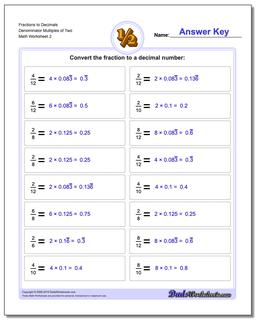PLEASE GO BACK AND USE THE BIG BLUE 'PRINT' BUTTON ON THE PAGE TO PRINT THE WORKSHEET CORRECTLY!Sorry for the trouble! The browser won't print the embedded worksheet PDF directly using the normal 'Print' command in the file menu, so you need to click the big 'Print' button to send just the worksheet and not the surrounding page to the printer.

# Math Worksheets: Fractions as Decimals: Fractions as Decimals: Fractions to Decimals Denominator Multiples of Two (Second Worksheet)## Fractions to Decimals Denominator Multiples of Two (Second Worksheet)

PropertyValue
DescriptionFractions to Decimals Denominator Multiples of Two: Drills for converting common fractions into decimal equivalents. (Second Worksheet)
Resource TypeWorksheet